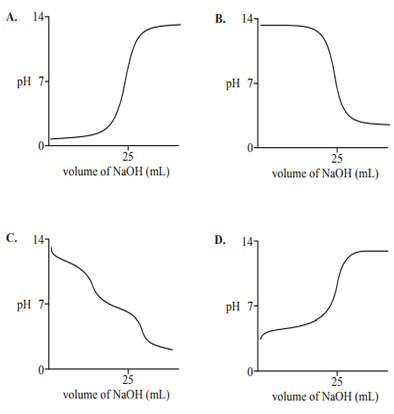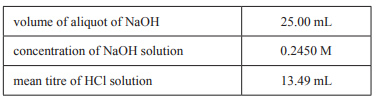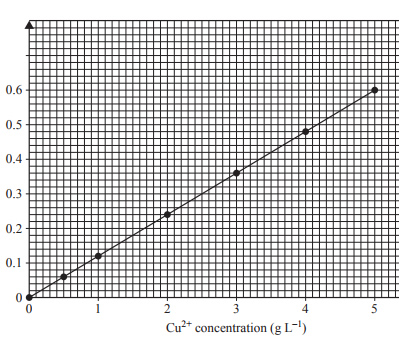Volumetric analysis (2016 VCE) 1) A solution of approximately 0.1 M benzoic acid, C6H5COOH, is titrated against a 0.1004 M solution of sodium hydroxide, NaOH. Which one of the following pH curves represents this titration?Solution2) A group of students was required to determine the concentration of a solution of hydrochloric acid, HCl, provided for a titration competition. In each titration, a 25.00 mL aliquot of a freshly standardised solution of 0.2450 M sodium hydroxide, NaOH, was pipetted into a conical flask and titrated against the HCl solution. An appropriate indicator was added. The experiment was repeated until three concordant results were obtained. The data for these titrations is shown in the following tablea) Based on these results, the concentration of HCl is A. 0.1322 M B. 0.4540 M C. 1.322 M D. 2.202 M Solutionb) The experimental value of the concentration of HCl obtained from these titrations was less than the actual value. Which one of these actions by the students most likely accounts for the lower than expected result? A. rinsing the burette with water B. rinsing the pipette with water C. rinsing the conical flask with water D. leaving the funnel in the top of the burette Solution4) The most suitable indicator for a titration of NaOH against benzoic acid, C6H5COOH, is A. bromophenol blue. B. methyl orange. C. thymol blue. D. phenol red. Solution5) Brass is an alloy of copper and zinc. To determine the percentage of copper in a particular sample of brass, an analyst prepared a number of standard solutions of copper(II) ions and measured their absorbance using an atomic absorption spectrometer (AAS). The calibration curve obtained is shown below.a) A 0.198 g sample of the brass was dissolved in acid and the solution was made up to 100.00 mL in a volumetric flask. The absorbance of this test solution was found to be 0.13 Calculate the percentage by mass of copper in the brass sample Solutionb) If the analyst had made up the solution of the brass sample to 20.00 mL instead of 100.00 mL, would the result of the analysis have been equally reliable? Why? Solutionc) Name another analytical technique that could be used to verify the result from part a. Solution